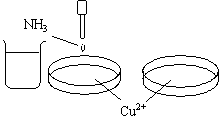## 18.8 Combined or Mixed Equilibria of Cu2+

Chemical Concept Demonstrated:  Complex-formation equilibrium

Demonstration:

 Each of the dishes contain Cu 2+ ion solution. Aqueous ammonia is added dropwise into the first dish. Excess ammonia is added into the first dish.Observations:

A precipitate forms as the ammonia is added dropwise into the dish.    Additional ammonia causes the precipitate to dissolve and a dark-blue solution is formed.

Explanations (including important chemical equations):

A light-blue colored precipitate of Cu(OH)2 is formed when aqueous ammonia is added to Cu 2+  ion solution.

Cu 2+  (aq) + 2 (OH)- (aq) <=> Cu(OH)2 (s)             Ksp = 2.2 x 10-20

In the presence of excess NH3, this equilibrium shifts to the left as the free Cu 2+ ion is sequestered from the solution as the Cu(NH3)4 2+ complex ion.

Cu 2+  (aq) + 4 NH3 (aq) <=> Cu(NH3)4 2+ (aq)    Ksp = 2.1 x 1013

The intensity of the absorption of light by the Cu(NH3)4 2+ complex appears to be orders of magnitude greater than the corresponding Cu(H2O)6 2+ complex.  The molar absorbances of these complexes, however, differ, roughly, only by a factor of four.  The difference between the intensities of the colors of these complexes can be explained by noting that the maximum wavelength for the Cu(H2O)6 2+ complex is about 800 nm, whereas the maximum wavelength for the Cu(NH3)4 2+ complex occurs at about 600 nm.  Since a larger portion of the absorption in the Cu(NH3)4 2+ complex is in the visible portion of the spectrum, the color appears considerably more intense.